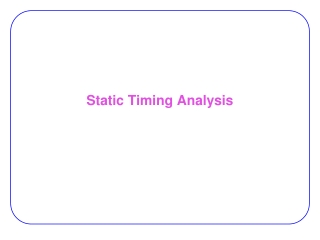DownloadDownload PresentationStatic Timing Analysis

# Static Timing Analysis

Télécharger la présentation## Static Timing Analysis

- - - - - - - - - - - - - - - - - - - - - - - - - - - E N D - - - - - - - - - - - - - - - - - - - - - - - - - - -
##### Presentation Transcript

1. Static Timing Analysis

2. Timing analysis • Need to know the clock frequency in the design process • Dynamic Timing Analysis: • Simulation: • Needs input vectors (not known during design process) •  Can miss an obscure performance-limiting path • Very slow •  Impractical in the loops of design phases

3. Timing analysis • Static Timing Analysis (STA): • Fast • Reasonably accurate measurement of timing • Simplified delay models • Calculates an upper bound on frequency • Conservative analysis •  Safe: guarantees that the design will function at least as fast as predicted • Delay characterization for cell libraries is clearly defined •  Forms an effective interface between the foundry and the design team

4. STA • Combinational circuits: Graph model: DAG • Vertices: • I/O pins of gates • s and t • Edges: • Connect each input of a gate to its output • Show maximum delay paths from the input pin to the output pin • Connects the output of each gate to the inputs of its fanout gates • Show interconnect delays • In case of combinational loop: • Many STA tools break the loop and analyze

5. STA

6. STA Timing Graph • Simplified graph 2 3 6 4 2 s 2 5 3

7. Sequential Circuits • Represented as: • a set of combinational blocks that lie between latches. • A timing graph may be constructed for each of these blocks • Inputs of graph: PIs + FF outputs • Outputs of graph: POs + FF inputs

8. Sequential Circuits • Algorithm for finding combinational blocks: • Construct a graph in which each vertex corresponds to a combinational element, • An undirected edge is drawn between a combinational element and the combinational elements that it fans out to • Sequential elements are left unrepresented • The connected components of this graph correspond to the combinational blocks

9. Gate Delay Models • Methods for calculating gate delay: • Look-up table method: • Each entry: delay under different capacitive loads and input transition times. • Good accuracy • But memory-intensive • Linear model: • Traditional: (k1 CL + k2): • k1: characterized slope • k2: intrinsic delay • Neglects the effect of the input transition time • k-factor equations: • Compact the table look-up by fitting function to • (k1 + k2CL)τin + k3CL3 + k4CL + k5

10. STA Algorithm • Traverse in topological order • Apply: 10

11. STA Algorithm 0 2 2 13 0 3 6 0 6 17 0 10 4 2 0 2 2 0 15 5 0 3 3 0 Wire delays ignored or added to the gate delays

12. STA: Critical Path • Critical path: • Trace back from the PO with largest arrival time • This is the output of last block on critical path • Identify the latest arriving input of the block • Identify the block that causes this transition • Repeat

13. Critical Path: Example 0 2 2 13 0 3 6 0 6 17 0 10 4 2 0 2 2 0 15 5 0 3 3 0

14. Required Arrival Times • If Timing constraints are specified: • For each node, must check if met or not • Useful to know which parts should be sped up • If ATnode > RTnode,  the path that the node lies on must be sped up

15. Required Arrival Times 13 15 • Consider delays as –ve • Apply the same procedure from output to inputs • Arrow directions reversed 2 18 13 3 9 3 6 20 3 Min(15,13) = 13 4 2 7 9 2 7 18 5 10 13 3 10

16. Slack • Slack of a node: • RTnode – ATnode • +ve slack s: •  Arrival time of that node can be increased by s w/o affecting the overall delay of circuit •  Potential to optimize power/area/…. • In FPGAs, >75% of nodes have ~ 50% slack

17. Incremental STA • In physical design loops: • A small change in module locations/connection paths • In design flow: • A small change in circuit • No need for full STA •  Incremental STA: • Event-driven procedure: • An event occurs when timing info at the input to the gate is changed • Only a small fraction of gates have their arrival times changed

18. d = 10 d = 10 d = 20 d = 20 False path False Paths • Many paths never occur • Considering them  pessimistic may waste resources • Assumption: • INV delay = 0 • Critical path: 40 • May try too hard to optimize it

19. False Paths • Solutions: • Automatic solutions: too complex to be practical • E.g. if inverter delay > 0 • In practice: • Designers knows functionalities best  Designer specifies

20. References • [Sapatnekar06] Sapatnekar, “Static Timing Analysis,” EDA for IC Implementation, Circuit Design, and Process Technology, 2006.